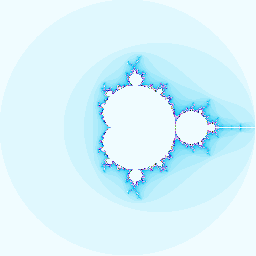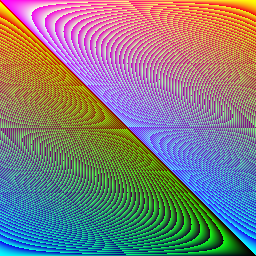#Coffee Space

Listen:

## Micro-CodePreview Image

### Background

These programs have been designed to be written on the back of a business card, with their output displayed on the front.

The following is the limitations on the design:

• Compile using strict C90
• Include comments for operation where possible
• Limitation on 80x25 characters

There are the following assumptions that are reasonable to make:

• Running a modern Linux OS
• Compiling with GCC

### Pattern Test

#### OutputPattern Test Image

#### Numbers

• With comments: 254 characters (not including newlines)
• Without comments: 201 characters (not including newlines)

#### Code

``````#include<stdio.h>/*gcc a.c; ./a.out; eog b.ppm*/
main(){int w=256,h=256,k;FILE*f=fopen("b.ppm","w");fprintf(f,"P6\n%d %d\n255\n"
,w,h);for(;--h>=0;){for(k=w;k>=0;--k){char c={h%256,(k*h)%256,k%256};fwrite(
c,1,3,f);}}fclose(f);}/*coffeespace.org.uk*/``````

#### Use

To compile:

``gcc a.c``

To run:

``./a.out``

To view:

``eog b.ppm``

This program scales well by changing what `w` and `h` equal, which are width and height respectively. Numbers that reflect `2^X` display nicer than others.

### Mandelbrot

#### OutputMandelbrot Image

#### Numbers

• Without comments: 291 characters (not including newlines)

#### Code

``````#include<stdio.h>
main(){int k=256,w,h=k,x,a=k/2;double b=a/2,i,j,y,z,t;FILE*f=fopen("b.ppm","w")
;fprintf(f,"P6\n%d %d\n255\n",k,k);for(;h--;)for(w=k;w--;){i=j=0;y=(w-a)/b;z=(h
-a)/b;x=k;for(;i*i+j*j<4&&x--;){t=i*i-j*j+y;j=2*i*j+z;i=t;}char c={x<<4,x<<2
,x};fwrite(c,1,3,f);}fclose(f);}``````

#### Use

To compile:

``gcc a.c``

To run:

``./a.out``

To view:

``eog b.ppm``

The image produced is really good looking and well detailed with `k = 256^X` where `X` is greater than 1. It has been tested with `k = 2^14 = 16385`, on a 3rd generation i5 processor, taking about 3 or 4 hours. This program certainly comes under the category of "embarrassingly parallel" and could benefit from multi-core processing, as little RAM and disk speed is used and pixels can be calculated independently.

### Difference

#### Output

FILE_1 (`t1.txt`)

``````Hello World!
1234567890
Difference
0123456789
hello``````

FILE_2 (`t2.txt`)

``````Hello World!
1234567890
difference
9876543210
hello
extra``````

Output

``````
t1.txt
Difference

t2.txt
difference


t1.txt
0123456789

t2.txt
9876543210


t2.txt
extra``````

#### Numbers

• Without comments: 383 characters (not including newlines)

#### Code

``````#include<stdio.h>
main(int c,char**v){int n=512,i=1,j,d=i,e=i;char a[n];char b[n];FILE*y=fopen(v[
1],"r");FILE*z=fopen(v,"r");for(;d&&e;++i){d=fgets(a,n,y);e=fgets(b,n,z);for
(j=-1;++j<n&&a[j]==b[j];)if(a[j]==0)j=n;if(j<n){char c={'[',(i/1000)+48,(i/
100%10)+48,(i/10%10)+48,(i%10)+48,']',0};puts(c);if(d){puts(v);puts(a);}if(e
){puts(v);puts(b);}}}fclose(y);fclose(z);}``````

#### Use

To compile:

``gcc a.c``

To run:

``./a.out FILE_1 FILE_2``

To view, read the terminal output.